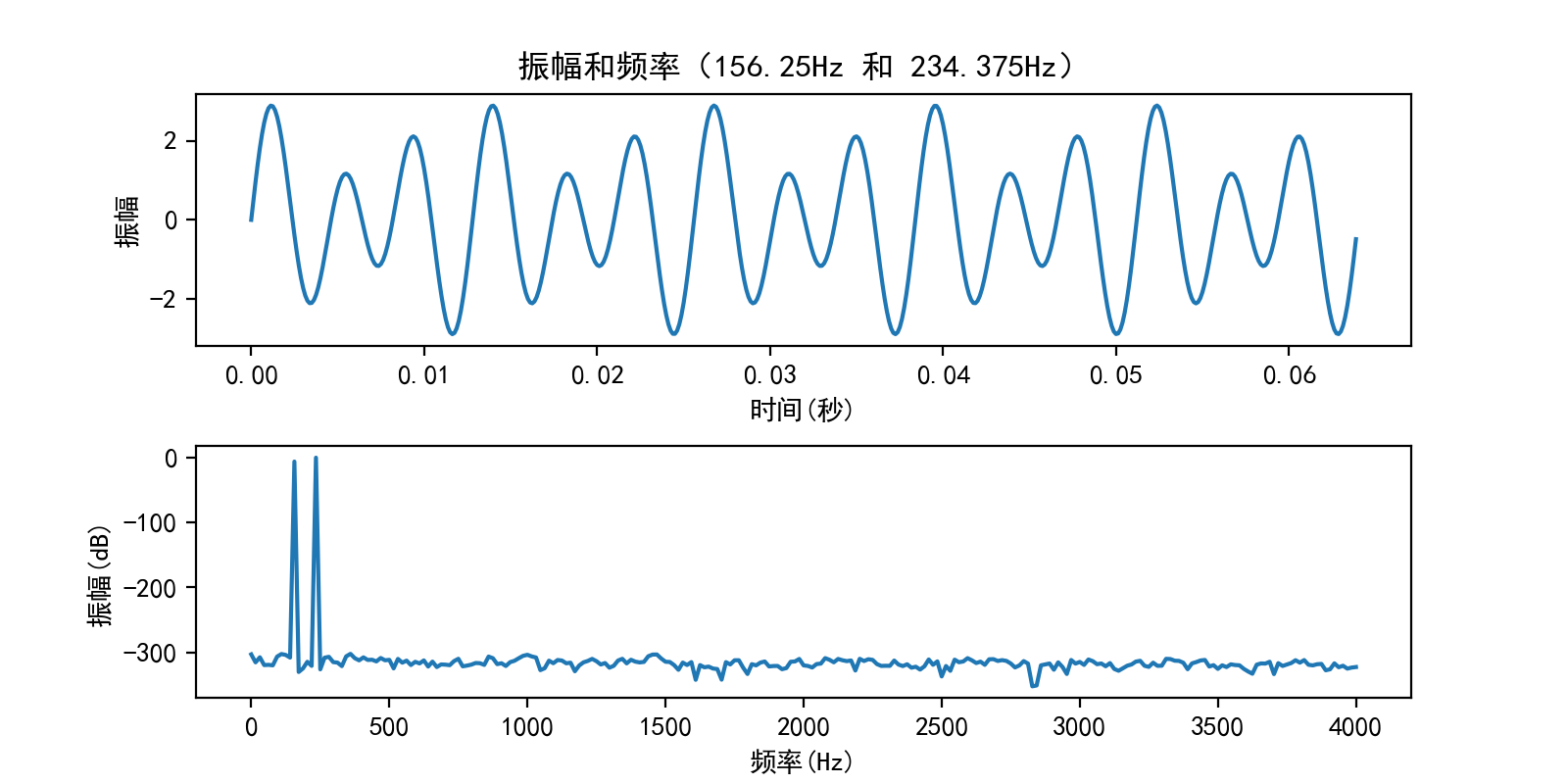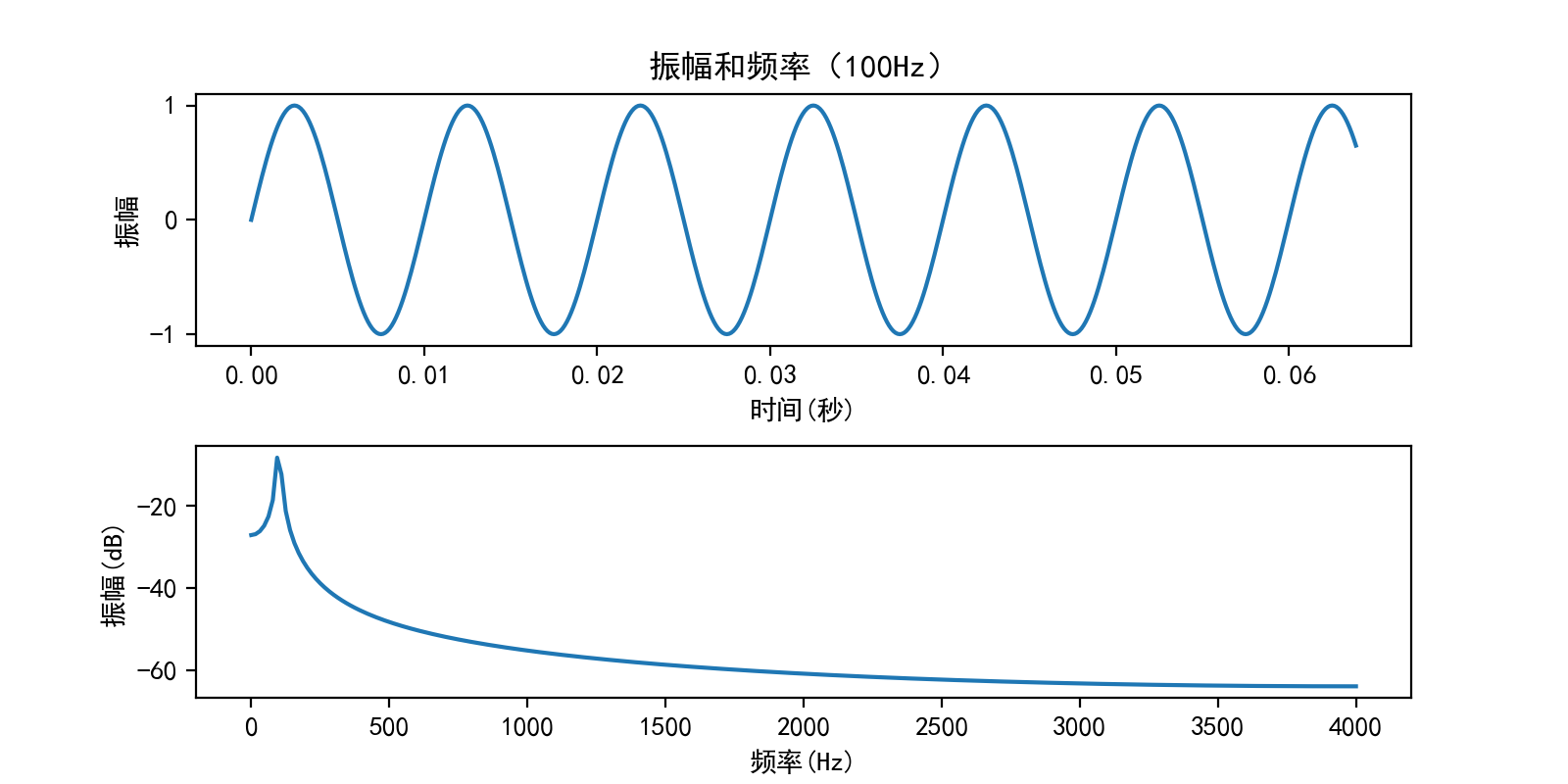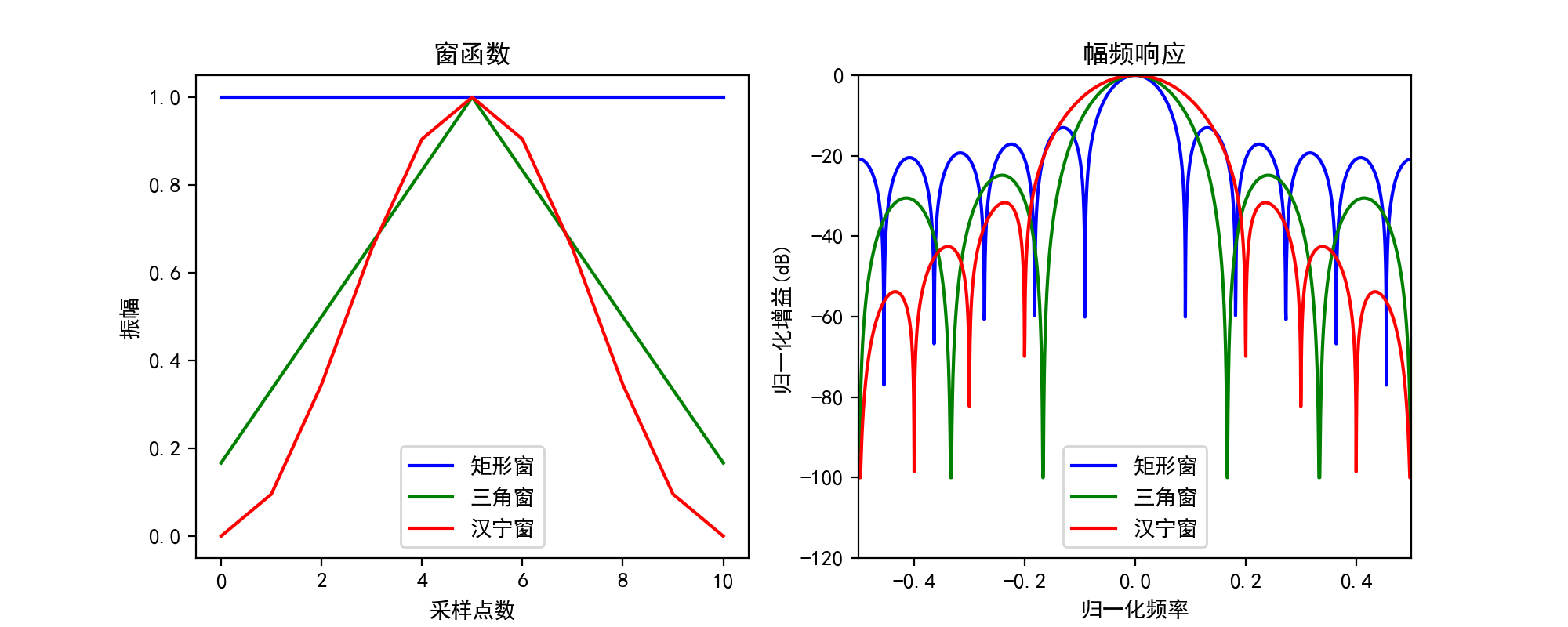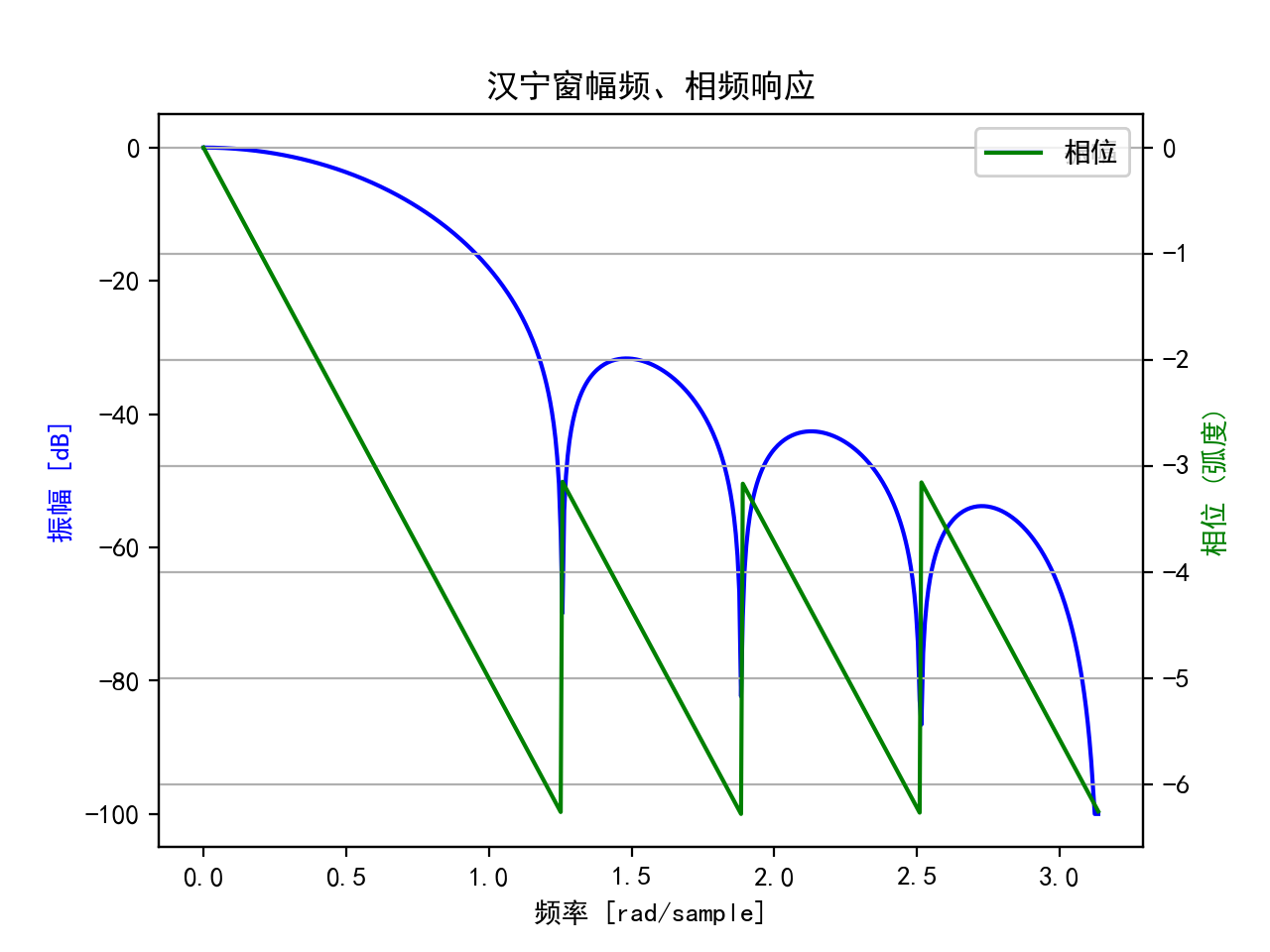17 May 2018

# python 之窗函数

• 周期截断是指截断后的信号为周期信号，这种截断不会导致频谱泄漏。
• 非周期截断是指截断后的信号不再是周期信号，哪怕原始信号本身是周期信号，这中截断会导致频谱泄漏。

• 主瓣越窄，频率分辨越准确
• 旁瓣越高，说明能量泄漏越严重
• 旁瓣衰减越慢，频谱拖尾越严重

## 周期截断

• 使用采样频率为 8000 Hz, 使用 N = 512 个采样点进行傅立叶变换（采样分辨率为 15.625 Hz)
• 令原始信号包含 156.25 和 234.375 两个正弦信号
• 不加窗（默认为矩形窗）对原始进行傅立叶变换，查看 FFT 变换是否有频谱泄漏
%matplotlib notebook
import numpy as np
import scipy.signal as signal
import matplotlib.pyplot as plt

sample_rate = 8000

fft_size  = 512

t = np.arange(0, 1.1, 1.0/sample_rate)

## 两个正弦信号，频率分辨为 156.25 和 234.375
x = np.sin(2*np.pi*156.25*t) + 2*np.sin(2*np.pi*234.375*t)

## 取 N 个采样点进行 FFT 变换
# rfft 函数的返回 N/2+1 个复数，分别表示从 0(Hz) ~ fs/2 频率分量
xs = x[:fft_size]
xf = np.fft.rfft(xs) / fft_size

## 生成频率和振幅(dB)
freqs = np.linspace(0, sample_rate/2, int(fft_size/2 + 1))
xfp = 20 * np.log10(np.clip(np.abs(xf), 1e-20, 1e1000))

## 画出波形及其频谱图
plt.figure(figsize=(8,4))
plt.subplot(211)
plt.plot(t[:fft_size], xs)
plt.xlabel("时间(秒)")
plt.ylabel("振幅")
plt.title("振幅和频率（156.25Hz 和 234.375Hz）")

plt.subplot(212)
plt.plot(freqs, xfp)
plt.xlabel("频率(Hz)")
plt.ylabel("振幅(dB)")

plt.show()## 非周期截断

## 100Hz 正弦信号，不是 15.625 倍数时，出现频谱泄漏
x = np.sin(2*np.pi*100*t)

## 取 N 个采样点进行 FFT 变换
# rfft 函数的返回 N/2+1 个复数，分别表示从 0(Hz) ~ fs/2 频率分量
xs = x[:fft_size]
xf = np.fft.rfft(xs) / fft_size

## 生成频率和振幅(dB)
freqs = np.linspace(0, sample_rate/2, int(fft_size/2+1))
xfp = 20 * np.log10(np.clip(np.abs(xf), 1e-20, 1e1000))

## 画出波形及其频谱图
plt.figure(figsize=(8,4))
plt.subplot(211)
plt.plot(t[:fft_size], xs)
plt.xlabel("时间(秒)")
plt.ylabel("振幅")
plt.title("振幅和频率（100Hz）")

plt.subplot(212)
plt.plot(freqs, xfp)
plt.xlabel("频率(Hz)")
plt.ylabel("振幅(dB)")

plt.show()100Hz 峰值处的幅值减小，泄漏到整个频带的其他谱线上。频谱图已经不能准确反映原始信号的频率了。

# 窗函数

%matplotlib notebook
import numpy as np
import scipy.signal as signal
import matplotlib.pyplot as plt
from scipy.fftpack import fft, fftshift

# 主瓣和旁瓣个数
win_size = 11
fft_size = 2048

# 生成矩形窗、三角窗、含宁窗数据
rect_win = np.ones(win_size)
triang_win = signal.triang(win_size)
hanning_win = signal.hanning(win_size)

# 计算三种窗的频率响应
rect_h = fft(rect_win, fft_size)
triang_h = fft(triang_win, fft_size)
hanning_h = fft(hanning_win, fft_size)

freqs = np.linspace(-0.5, 0.5, fft_size)

def a2db(amp):
return 20 * np.log10(np.clip(np.abs(fftshift(amp / abs(amp).max())), 1e-5, 1e100))

plt.figure(figsize=(10,4))

plt.subplot(121)
plt.xlabel('采样点数')
plt.ylabel('振幅')
plt.title('窗函数')
plt.plot(rect_win, color='b', label='矩形窗')
plt.plot(triang_win, color='g', label='三角窗')
plt.plot(hanning_win, color='r', label='汉宁窗')
plt.legend()

plt.subplot(122)
plt.title('幅频响应')
plt.xlabel('归一化频率')
plt.ylabel('归一化增益(dB)')
plt.axis([-0.5, 0.5, -120, 0])
plt.plot(freqs, a2db(rect_h), color='b', label='矩形窗')
plt.plot(freqs, a2db(triang_h), color='g', label='三角窗')
plt.plot(freqs, a2db(hanning_h), color='r', label='汉宁窗')
plt.legend()

plt.show()%matplotlib notebook
import numpy as np
import scipy.signal as signal
import matplotlib.pyplot as plt

win_size = 11

## 矩形窗
hann_window = signal.hanning(win_size)
hann_w, hann_h = signal.freqz(rect_window)
db = 20 * np.log10(np.clip(np.abs(hann_h/abs(hann_h).max()), 1e-5, 1e100))

plt.figure()
plt.title('汉宁窗幅频、相频响应')
plt.plot(hann_w, db, color='b', label='振幅')
plt.ylabel('振幅 [dB]', color='b')
plt.legend()

plt.twinx()
rect_angles = np.unwrap(np.angle(rect_h))
plt.plot(rect_w, rect_angles, color='g', label='相位')
plt.ylabel('相位 (弧度)', color='g')
plt.grid()
plt.axis('tight')
plt.legend()
plt.show()